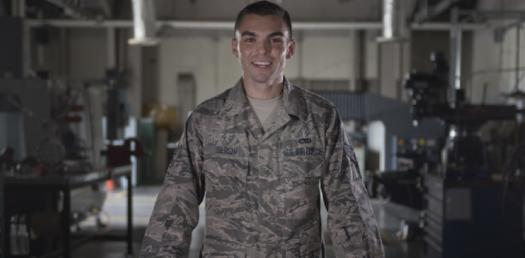# 2A751 : Aircraft Metals Technology Journeyman! Trivia Quiz

101 Questions | Total Attempts: 129Settings.

• 1.
When drafting, from where should the light fall on the drawing surface?
• A.

Directly over the head of the drafter

• B.

Pointed in the direction of the drafter

• C.

Over the left shoulder of a left handed drafter

• D.

Over the left shoulder of a right handed drafter

• 2.
Covering the drawing board with vinyl or heavy paper.
• A.

Helps light diffuse on the paper

• B.

Keeps the drafting paper from slipping

• C.

Keeps the drafter from getting splinters

• D.

Helps keep the table from being damaged

• 3.
One quality of vellum or tracing paper is
• A.

Wrinkle resistance

• B.

Heavy construction

• C.

Moisture resistance

• D.

Ease of reproduction

• 4.
To store blueprints or mechanical drawings, you should
• A.

Hang them up

• B.

Store them flat

• C.

Keep them cool

• D.

Fold them neatly

• 5.
Basic drafting equipment consists of
• A.

A compass, layout die, and drafting instruments

• B.

A drawing board, T square, and drafting instruments

• C.

Hermaphrodite calipers, a drawing board, and layout die

• D.

Drafting instruments, a scribe, and hermaphrodite calipers

• 6.
To keep your T square from warping, you should
• A.

Lay it flat

• B.

Stand it up

• C.

Oil it regularly

• D.

• 7.
When you need to quickly draw angles of other than multiples of 15 degrees, a good tool to select is
• A.

A compass

• B.

A protractor

• C.

An architect's scale

• D.

• 8.
The purpose of an engineer's or architect's scale is to
• A.

Construct lines specifically for an architect

• B.

Construct lines specifically for an engineer

• C.

Reduce or enlarge drawings based on the scale

• D.

Reduce or enlarge drawings based on the print size

• 9.
The engineer's scale divides inches into
• A.

Decimals

• B.

Fractions

• C.

Centimeters

• D.

Millimeters

• 10.
The scale used the most often in drafting, is the
• A.

Vernier scale

• B.

Six inch scale

• C.

Engineer's scale

• D.

Architect's scale

• 11.
The compass, least likely to spring out of shape during use, is the
• A.

Variable compass

• B.

End bow compass

• C.

Side bow compass

• D.

Center bow compass

• 12.
If you need to increase the radius of a circle when using the fraction head compass, select
• A.

Extension bars

• B.

A large divider

• C.

Trammel points

• D.

Side bow attachment

• 13.
The tool used to transfer measurements and distances is
• A.

Dividers

• B.

Large calipers

• C.

A bow compass

• D.

A trammel point set

• 14.
When  you use dividers to step off equal spaces on a line, you
• A.

Rotate them toward the drawing border

• B.

Step them in a counter clockwise direction

• C.

Alternate clockwise an counter clockwise steps

• D.

Rotate them ina continuous clockwise direction

• 15.
You can reduce the time you do repetitive drafting jobs by using
• A.

A drafting set

• B.

A bow compass

• C.

A drafting template

• D.

• 16.
If you use a heavy coating of layout dye on your workpiece, you'll have
• A.

Ragged and uneven lines

• B.

Thin, fine lines for layout

• C.

Distinct, even layout lines

• D.

A dark surface for layout lines

• 17.
A good application of the small rule with holder is measuring
• A.

Internal recesses

• B.

Cylindrical surfaces

• C.

Flat parallel surfaces

• D.

Gear tooth peripheries

• 18.
When  you construct a figure using geometric construction, use a
• A.

Pencil, a compass, and a straightedge

• B.

Pencil, a divider set, and a drafting set

• C.

Drafting pen, a drafting set, and a ruler

• D.

Straightedge, a drafting set, and a divider

• 19.
When you need to divide an area into equal spaces, use a scale that
• A.

Spans slightly less than the space you want to divide

• B.

Spans slightly more than the space you want to divide

• C.

Is any size that permits you to measure the top boundary line

• D.

Is any length as long as it's shorter that the top boundary line

• 20.
When constructing a perpendicular bisector, the method most accurate is
• A.

Using geometric construction

• B.

Laying out an arc tangent first

• C.

Laying out a right triangle first

• D.

Using a T square and a triangle

• 21.
Which is not true of using arcs?
• A.

Lends speed

• B.

Is not accurate

• C.

Lends accuracy

• D.

Eliminates the use of a scale

• 22.
Whe constructing circles and arcs on drawings, first draw
• A.

The straight lines

• B.

The circles or arcs

• C.

Bisectors of circles

• D.

Straight line tangents

• 23.
When constructing a straight line, equal to an arc, set  the dividers to divide the
• A.

Line into two parts

• B.

Arc into equal parts

• C.

Line into equal parts

• D.

Arc into unequal parts

• 24.
When you construct plane figures, you're showing
• A.

One dimensional objects, like a box

• B.

Two dimensional objects, like a cube

• C.

Three dimensional objects, like a ball

• D.

Four dimensional objects, like a sphere

• 25.
A pictoral view of an object is given by an
• A.

Oblique projection

• B.

Isometric projection

• C.

Orthographic projection

• D.

Axonometric projection

Related TopicsBack to top